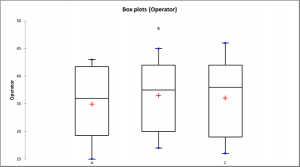# Gage RR / Cuantitativos

Gage Repeatability and Reproducibility assesses a measurement process. Gage R&R for quantitative variables available in Excel with the XLSTAT software.## What is Gage R&R for quantitative variables (Measurement System Analysis)

Measurement System Analysis (MSA) or Gage R&R (Gage Repeatability and Reproducibility) is a method to control and assess a measurement process. It is useful to determine which sources are responsible for the variation of the measurement data. Variability can be caused by the measurement system, the operator or the parts. Gage R&R applied to quantitative measurements is based on two common methods: ANOVA and R control charts.

The word gage (or gauge) refers to the fact that the methodology is aimed at validating instruments or measurement methods. For quantitative data, two characteristics are of interest:

• Repeatability: A measurement is "repeatable" if the measures taken by a given operator for the same object (product, unit, part, or sample, depending of the field of application) repeatedly, do not vary above a given threshold. If the repeatability of a measurement system is not satisfactory, one should question the quality of the measurement system, or train the operators that do not obtain repeatable results if the measurement system does not appear to be responsible for the high variability.
• Reproducibility: A measurement is "reproducible" if the measures obtained for a given object (product, unit, part, or sample, depending of the field of application) by several operators do not vary above a given threshold. If the reproducibility of a measurement system is not satisfactory, one should train the operators so that their results are more homogeneous.

The goal of a Gage R&R analysis is to identify the sources of variability and to take the necessary actions to reduce them if necessary.

## Gage R&R for quantitative variables in XLSTAT

The Gage R&R for quantitative variables XLSTAT tools should be used to control and validate your measurement method and measurement systems, in the case where you have several quantitative measures taken by one or more operators on several parts.

When the measures are quantitative data, two alternative methods are available for Gage R&R analysis. This first is based on analysis of variance (ANOVA) and the second on R control charts (Range and average).

## ANOVA to compute Gage R&R for quantitative variables in XLSTAT

When the ANOVA model is used in R&R analysis, one can statistically test whether the variability of the measures is related to the operators, and/or to the parts being measured themselves, and/or to an interaction between both (some operators might give for some parts significantly higher or lower measures), or not. Two designs are available when doing gage R&R analysis: the crossed design (balanced) and the nested design. XLSTAT includes as of today the crossed and designs.

## R charts to compute Gage R&R for quantitative variables

While less powerful than the ANOVA method, the Gage R&R analysis based on Range and Average analysis, is easy to compute and produces control charts (R charts). As the ANOVA method, it allows to compute the repeatability and the reproducibility of the measurement process. To use this method you need to have several parts, operators and repetitions (typically 10 parts, 3 operators, and 2 repetitions).

## Indicators for Gage R&R for quantitative variables

XLSTAT offers several indicators derived from the variances to describe the measurement system.

• Study variation: The study variation for the different sources is calculated as product of the corresponding standard deviation of the source and the used defined factor k Sigma: Study variation = k*Ŝ
• % tolerance: The tolerance in percent is defined as the ratio of the variance in the study and the user defined tolerance: % tolerance = Study variation / tolerance
• The process sigma in percent is defined as ratio of the standard deviation of the source and the user defined historic process sigma: % process = standard deviation of the source / process sigma
• Precision to tolerance ratio (P/T): P / T = k* ŜR&R² / tolerance
• Rho P (Rho Part): ρpart = Ŝpart² / Ŝ²
• Rho M: ρM = ŜR&R² / Ŝ²
• Signal to noise ratio (SNR): SNR = [2 ρpart / (1- ρpart)]1/2
• Discrimination ratio (DR): DR = (1 + ρpart) / (1 - ρpart)
• Bias: Bias = µmeasurements- target
• Bias in percent: Bias % = µmeasurements- target / tolerance
• Resolution: Resolution = Bias + 3* ŜR&R²### analice sus datos con xlstat

prueba gratuita de 14 días

Incluido en